4430.0 - Disability, Ageing and Carers, Australia: Summary of Findings, 2009
ARCHIVED ISSUE Released at 11:30 AM (CANBERRA TIME) 16/12/2010
Page tools:Print All

TECHNICAL NOTE DATA QUALITY

RELIABILITY OF THE ESTIMATES

1 Since the estimates in this publication are based on information obtained from occupants of a sample of dwellings, they are subject to sampling variability. That is, they may differ from those estimates that would have been produced if all dwellings had been included in the survey. One measure of the likely difference is given by the standard error (SE), which indicates the extent to which an estimate may have varied by chance because only a sample of dwellings was included. There are about two chances in three (67%) that a sample estimate will differ by less than one SE from the number that would have been obtained if all dwellings had been included, and about 19 chances in 20 (95%) that the difference will be less than two SEs.

2 Another measure of the likely difference is the relative standard error (RSE), which is obtained by expressing the SE as a percentage of the estimate.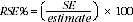3 RSEs for estimates from the 2009 SDAC were calculated using the Jackknife method of variance estimation. This involves the calculation of 60 'replicate' estimates based on 60 different sub-samples of the original sample. The variability of estimates obtained from these sub-samples is used to estimate the sample variability surrounding the main estimate.

4 Tables 1, 2 and 4 contain time series estimates from the 2009 SDAC and 2003 SDAC. The spreadsheet datacubes associated with the current edition of Disability, Ageing and Carers, Australia: Summary of Findings (cat. no. 4430.0) contain directly calculated RSEs for the 2009 estimates. However, the RSEs for the 2003 estimates were calculated using a previous statistical SE model. This is detailed in Disability, Ageing and Carers, Australia: Summary of Findings, 2003 (cat. no. 4430.0) which is available on the ABS website <www.abs.gov.au>. While the direct method is more accurate, the difference between the two is usually not significant for most estimates.

5 Estimates (numbers and percentages) with RSEs less than 25% are considered sufficiently reliable for most purposes. However, estimates with larger RSEs are included and preceded by an asterisk (e.g. *3.9) to indicate they are subject to high SEs and should be used with caution. Estimates with RSEs greater than 50% are preceded by a double asterisk (e.g. **1.2) to indicate that they are considered too unreliable for general use.

CALCULATION OF STANDARD ERRORS

6 Standard errors can be calculated using the estimates and the corresponding RSEs. For example, Table 1 shows the estimated number of females in 2009 who had a disability was 2,063,100. The RSE Table corresponding to the estimates in Table 1 (see Relative Standard Errors for Table 1 in the 'Relative Standard Error' section at the end of these Technical Notes) shows the RSE for this estimate is 1.2%. The SE is calculated by: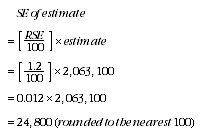7 Therefore, there are about two chances in three that the value that would have been produced if all dwellings had been included in the survey will fall within the range 2,038,300 to 2,087,900 and about 19 chances in 20 that the value will fall within the range 2,013,500 to 2,112,700. This example is illustrated in the diagram below.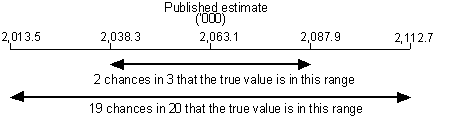PROPORTIONS AND PERCENTAGES

8 Proportions and percentages formed from the ratio of two estimates are also subject to sampling error. The size of the error depends on the accuracy of both the numerator and the denominator. A formula to approximate the RSE of a proportion is given below. The formula is only valid when the numerator is a subset of the denominator: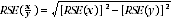9 As an example, using the 2009 estimates from Table 1, of the 4,026,200 persons who had a disability, 2,063,100 are females or 51.2%. The RSE for 2,063,100 is 1.2% and the RSE for 4,026,200 is 1.0% (see Relative Standard Errors for Table 1 in the 'Relative Standard Error' section at the end of these Technical Notes). Applying the above formula, the RSE for the proportion of females who had a disability is: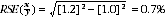10 Therefore, the SE for the proportion of persons who had a disability and were female, is 0.4 percentage points (=0.7/100 x 51.2). Hence, there are about two chances in three that the proportion of females who had a disability is between 50.8% and 51.6%, and 19 chances in 20 that the proportion is between 50.4% and 52.0%.

DIFFERENCES

11 Published estimates may also be used to calculate the difference between two survey estimates (of numbers or percentages). Such an estimate is subject to sampling error. The sampling error of the difference between two estimates depends on their SEs and the relationship (correlation) between them. An approximate SE of the difference between two estimates (x-y) may be calculated by the following formula: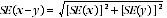12 While this formula will only be exact for differences between separate and uncorrelated characteristics or sub-populations, it is expected to provide a good approximation for all differences likely to be of interest in this publication.

SIGNIFICANCE TESTING

13 A statistical significance test for any of the comparisons between estimates can be performed to determine whether it is likely that there is a difference between the corresponding population characteristics. The standard error of the difference between two corresponding estimates (x and y) can be calculated using the formula in paragraph 11. This standard error is then used to calculate the following test statistic: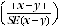14 If the value of this test statistic is greater than 1.96 then there is evidence of a statistically significant difference (at the 5% level) in the two estimates with respect to that characteristic. This statistic corresponds to a 95% confidence interval of the difference. Otherwise, it cannot be stated with confidence that there is a real difference between the population with respect to that characteristic.

15 The selected tables in this publication that show the results of significance testing are annotated to indicate where the estimates are significantly different from each other. In all other tables which do not show the results of significance testing, users should take account of RSEs when comparing estimates for different populations.

AGE STANDARDISATION

16 For this publication the direct age standardisation method was used. The standard population used was the 30 June 2001 Estimated Resident Population. Estimates of age-standardised rates were calculated using the following formula: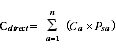where:
n = number of age categories
Cdirect = the age-standardised rate for the population of interest
a = the age categories that have been used in the age standardisation
Ca = the estimated rate for the population being standardised in age category a
Psa = the proportion of the standard population in age category a.

17 The age categories used in the standardisation for this publication were 0 to 4 years, 5 to 14 years, 15 to 24 years, 25 to 34 years, 35 to 44 years, 45 to 54 years, then five-year groups to 90 years and over.

RELATIVE STANDARD ERROR

18 Limited publication space does not allow for the separate indication of the SEs and/or RSEs of all the estimates in this publication, and as a result only RSEs for Table 1 are included. However, RSEs for all tables are available free-of-charge on the ABS website <www.abs.gov.au>, released in spreadsheet format as an attachment to this publication.

 RELATIVE STANDARD ERRORS FOR TABLE 12003 2009RSE of estimate RSE of proportion RSE of estimate RSE of proportion Age group (years) % % % % Males 0-4 15.3 14.9 10.6 10.6 5-14 6.4 6.1 5.0 5.0 15-24 7.4 7.1 6.9 6.9 25-34 6.3 6.0 5.2 5.3 35-44 5.7 5.3 4.3 4.3 45-54 4.8 4.3 3.5 3.5 55-59 6.5 5.5 3.8 3.8 60-64 6.3 4.9 3.8 3.8 65-69 6.9 5.3 3.6 3.6 70-74 6.9 5.0 3.2 3.2 75-79 7.1 4.5 3.3 3.3 80-84 8.1 4.3 3.2 3.0 85-89 11.8 5.9 4.9 3.4 90 and over 18.1 5.3 11.1 4.2 Total 1.7 1.6 1.2 1.2 Total, age standardised(a) . . 1.6 . . 1.2 Females 0-4 17.0 16.6 12.6 12.6 5-14 8.5 8.2 6.2 6.2 15-24 7.5 7.2 6.4 6.4 25-34 7.0 6.7 4.8 4.8 35-44 5.8 5.4 4.2 4.2 45-54 4.8 4.4 3.6 3.6 55-59 6.2 5.2 3.7 3.7 60-64 6.7 5.4 3.5 3.5 65-69 7.1 5.7 4.3 4.3 70-74 6.5 4.7 3.8 3.8 75-79 6.4 4.3 3.2 3.2 80-84 6.8 3.9 3.1 3.1 85-89 8.5 3.9 3.4 2.1 90 and over 10.5 2.9 5.4 2.2 Total 1.7 1.5 1.2 1.2 Total, age standardised(a) . . 1.5 . . 1.2 Persons 0-4 11.5 11.3 8.3 8.3 5-14 5.1 4.9 4.0 4.0 15-24 5.2 5.0 5.3 5.3 25-34 4.6 4.4 3.8 3.8 35-44 4.0 3.7 3.5 3.5 45-54 3.3 3.0 2.7 2.7 55-59 4.4 3.8 2.8 2.8 60-64 4.5 3.6 2.7 2.7 65-69 4.9 3.9 2.7 2.7 70-74 4.7 3.4 2.5 2.5 75-79 4.7 3.1 2.3 2.3 80-84 5.1 2.9 2.4 2.3 85-89 6.9 3.3 2.9 1.9 90 and over 9.1 2.6 5.3 2.2 Total 1.1 1.0 1.0 1.0 Total, age standardised(a) . . 1.0 . . 1.0 . . not applicable (a) Age standardised to the estimated resident population at 30 June 2001.